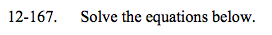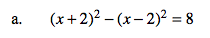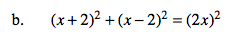### Home > A2C > Chapter 12 > Lesson 12.4.1 > Problem12-167

12-167.
1. Solve the equations below. Homework Help ✎

1. (x + 2)2 − (x − 2)2 = 8

2. (x + 2)2 + (x − 2)2 = (2x)2Expand the binomials before distributing the minus (−).

x = 1Square each term first.